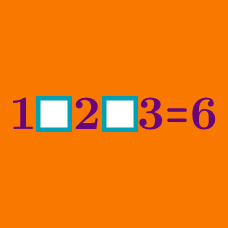Logic

# Addition and subtraction only - Operator Search

$1 \; \square \; 2 \; \square \; 4 \; \square \; 8 < 0$

Which of the following series of signs are appropriate for the three boxes above?

If you fill in the blanks with $$+$$ and/or $$-$$ only, how many $$+$$ do you need to use to make this equation true?

$2 \; \square \; 5 \; \square \; 6 \; \square \; 7 = 10$

$1 \; \square \; 1 \; \square \; 1 \; \square \; 1$

If we fill in the above blanks using only the operators $$+$$ and/or $$-$$, which of the following $$\color{red} { \text{cannot} }$$ be a resultant number?

$9 \; \square \; 7 \; \square \; 5 \; \square \; 3$

If we fill in the above blanks using only the operators $$+$$ and/or $$-$$, the resultant number will always be an $$\text{__________}$$.

$2 \; \square \; 3 \; \square \; 3\; \square \; 6 = 2$

Which of the following series of signs are $$\color{red}{\text{not}}$$ appropriate for the three boxes above?

×

Problem Loading...

Note Loading...

Set Loading...